#### 期刊菜单

Research on Finite-Time Sliding Mode Control Algorithm for Vehicle System
DOI: 10.12677/DSC.2023.122006, PDF , HTML, XML, 下载: 154  浏览: 361

Abstract: This paper mainly studies the finite time sliding mode control of three-degree-of-freedom vehicle systems. For trajectory tracking, the performance of three sliding mode control schemes is discussed and compared from the aspects of finite arrival time, singularity and the chattering phenomenon of control input. Firstly, in each section, three kinds of sliding mode surfaces and controller designs of linear sliding mode control, traditional terminal sliding mode control and non- singular terminal sliding mode control are presented respectively. Then, the stability of ship system is analyzed by Lyapunov stability theorem and the validity of the theorem is proved by finite-time theory. Next, the finite arrival time of each control method is analyzed. Finally, the vehicle trajectory tracking and the performance comparison of the three sliding mode control methods are realized by numerical simulation to verify the accuracy of the mentioned theory.

1. 引言

2. 问题描述及预备知识

$\stackrel{˙}{\eta }=R\left(\psi \right)v$

$M\stackrel{˙}{v}+C\left(\nu \right)v+D\left(\nu \right)\nu =\tau +{d}_{0}$ (1)

$R\left(\psi \right)=\left[\begin{array}{ccc}\mathrm{cos}\left(\psi \right)& -\mathrm{sin}\left(\psi \right)& 0\\ \mathrm{sin}\left(\psi \right)& \mathrm{cos}\left(\psi \right)& 0\\ 0& 0& 1\end{array}\right]$$M=\left[\begin{array}{ccc}m-{X}_{\stackrel{˙}{u}}& 0& 0\\ 0& m-{Y}_{\stackrel{˙}{v}}& m{x}_{g}-{Y}_{\stackrel{˙}{r}}\\ 0& m{x}_{g}-{N}_{\stackrel{˙}{v}}& {I}_{z}-{N}_{\stackrel{˙}{r}}\end{array}\right]$

$C\left(v\right)=\left[\begin{array}{ccc}0& 0& {c}_{13}\left(v\right)\\ 0& 0& {c}_{23}\left(v\right)\\ -{c}_{13}\left(v\right)& -{c}_{23}\left(v\right)& 0\end{array}\right]$$D\left(v\right)=\left[\begin{array}{ccc}{d}_{11}\left(v\right)& 0& 0\\ 0& {d}_{22}\left(v\right)& {d}_{23}\left(v\right)\\ 0& {d}_{32}\left(v\right)& {d}_{33}\left(v\right)\end{array}\right]$

$S\left(r\right)=\left(\begin{array}{rrr}\hfill 0& \hfill -r& \hfill 0\\ \hfill r& \hfill 0& \hfill 0\\ \hfill 0& \hfill 0& \hfill 0\end{array}\right)$m为船体的质量， ${I}_{Z}$ 为船舶所受转动的影响， ${X}_{\left(·\right)}$${Y}_{\left(·\right)}$${N}_{\left(·\right)}$ 表示水动力阻尼系数，

$\begin{array}{c}{\stackrel{˙}{\eta }}_{2}=RS\left(r\right)v+R{M}^{-1}\left(-{C}_{0}\left(v\right)v-{D}_{0}\left(v\right)\nu +\tau -\Delta C\left(v\right)v-\Delta D\left(v\right)v+{d}_{0}\left(t\right)\right)\\ =S\left(v\right){\eta }_{2}-R\left({\eta }_{1}\right){M}^{-1}\left(C\left(v\right)+D\left(v\right)\right)v+R\left(\psi \right){M}^{-1}\tau +d\\ =f\left(\eta \right)+g\left(\eta \right)\tau +d\end{array}$ (2)

$\left\{\begin{array}{l}{\stackrel{˙}{\eta }}_{1}={\eta }_{2}\\ {\stackrel{˙}{\eta }}_{2}=f\left(\eta \right)+g\left(\eta \right)\tau +d\end{array}$ (3)

$\left\{\begin{array}{l}{\stackrel{˙}{\eta }}_{d1}={\eta }_{d2}\\ {\stackrel{˙}{\eta }}_{d2}={F}_{d}\left({\eta }_{d},{\tau }_{d}\right)\end{array}$ (4)

$\left\{\begin{array}{l}{\stackrel{˙}{\chi }}_{1}={\chi }_{2}\\ {\stackrel{˙}{\chi }}_{2}=f\left(\eta \right)-{F}_{d}\left({\eta }_{d},{\tau }_{d}\right)+g\left(\eta \right)\tau +d\end{array}$ (5)

$x=f\left(x\right),\text{}f\left(0\right)=0,\text{}x\left(0\right)={x}_{0}$

$\stackrel{˙}{V}\left(x\right)\le -q{V}^{\alpha }\left( x \right)$

$T\left({x}_{0}\right)\le \frac{1}{q\left(1-\alpha \right)}{V}^{1-\alpha }\left( x 0 \right)$

3. 主要研究成果

3.1. 基于传统线性滑模的控制研究

$s={C}_{0}{\chi }_{1}+{\chi }_{2}$ (6)

$\tau =g{\left(\eta \right)}^{-1}\left(-{C}_{0}{\chi }_{2}-f\left(\eta \right)+{F}_{d}-ksign\left(s\right)\right)$ (7)

$\begin{array}{c}\stackrel{˙}{s}={C}_{0}{\chi }_{2}+f\left(\eta \right)-{F}_{d}\left({\eta }_{d},{\tau }_{d}\right)+g\left(\eta \right)\tau +d\\ ={C}_{0}{\chi }_{2}+f\left(\eta \right)-{F}_{d}-{C}_{0}{\chi }_{2}-f\left(\eta \right)+{F}_{d}-ksign\left(s\right)+d\\ =d-ksign\left( s \right)\end{array}$

$\stackrel{˙}{V}={s}^{\text{T}}\left(d-ksign\left(s\right)\right)\le \left({l}_{d}-k\right)‖s‖=-{\mu }_{0}{V}^{\frac{1}{2}}$ (8)

3.2. 基于终端滑模的控制研究

$s={\chi }_{2}+\delta {\chi }_{1}^{\frac{q}{p}}$ (9)

$\tau =-g{\left(\eta \right)}^{-1}\left(f\left(\eta \right)-{F}_{d}+\delta \frac{q}{p}{\chi }_{1}^{\frac{q}{p}-1}{\chi }_{2}+\left({l}_{d}+{\mu }_{1}\right)sign\left(s\right)\right)$ (10)

$\begin{array}{c}\stackrel{˙}{s}={\stackrel{˙}{\chi }}_{2}+\delta \cdot \frac{q}{p}{\chi }_{1}^{\frac{q}{p}-1}{\chi }_{2}\\ =f\left(\eta \right)-{F}_{d}+g\left(\eta \right)\tau +d+\delta \frac{q}{p}{\chi }_{1}^{\frac{q}{p}-1}{\chi }_{2}\end{array}$

$\begin{array}{c}\stackrel{˙}{s}=f\left(\eta \right)-{F}_{d}-f\left(\eta \right)+{F}_{d}-\delta \frac{q}{p}{\chi }_{1}^{\frac{q}{p}-1}{\chi }_{2}-\left({l}_{d}+{\mu }_{1}\right)sign\left(s\right)+d+\delta \frac{q}{p}{\chi }_{1}^{\frac{q}{p}-1}{\chi }_{2}\\ =d-\left({l}_{d}+{\mu }_{1}\right)sign\left(s\right)\end{array}$ (11)

$\begin{array}{c}\stackrel{˙}{V}={s}^{\text{T}}\left(d-\left({l}_{d}+{\mu }_{1}\right)sign\left(s\right)\right)\\ \le \left(‖d‖-{l}_{d}-{\mu }_{1}\right)‖s‖\\ \le -{\mu }_{1}‖s‖=-{\mu }_{1}{V}^{\frac{1}{2}}\end{array}$ (12)

$s\left({t}_{1}\right)-s\left(0\right)=±{\mu }_{1}{t}_{1}$

${t}_{1}=|\frac{s\left(0\right)}{{\mu }_{1}}|=\frac{|s\left(0\right)|}{{\mu }_{1}}$

${\chi }_{2}+\delta {\chi }_{1}^{q/p}={\stackrel{˙}{\chi }}_{1}+\delta {\chi }_{1}^{q/p}=0$ (13)

${\int }_{{x}_{1}\left({t}_{1}\right)}^{0}{\chi }_{1}^{-q/p}\text{d}{\chi }_{1}={\int }_{{t}_{1}}^{{t}_{1}+{t}_{2}}-\delta \text{d}t$

$-\frac{p}{p-q}{\chi }_{1}^{1-\frac{q}{p}}\left({t}_{1}\right)=-\delta {t}_{2}$

${t}_{2}=\frac{p}{\delta \left(p-q\right)}{|{\chi }_{1}\left({t}_{1}\right)|}^{1-\frac{q}{p}}$ (14)

TSM控制器(10)中的第三项包含 ${\chi }_{1}^{q/p-1}{\chi }_{2}$ ，其中 $q/p-1<0$ 。因此，当 ${\chi }_{1}=0$ ，且 ${\chi }_{2}\ne 0$ 时，会发生奇异问题，奇异点的存在会使得控制输入在某时刻趋于无穷大造成控制系统的不稳定性。由式(13)可知，当系统轨迹已经到达了滑模面之后，此项 ${\chi }_{1}^{q/p-1}{\chi }_{2}$ 就等同于 ${\chi }_{1}^{\left(2q-p\right)/p}$ 。从理论上讲，此时并不会发生奇异问题，但是实际工程中会存在不确定因素以及计算误差，无法保证系统状态始终保持在滑动模式中，特别是在平衡点附近( ${\chi }_{1}=0$${\chi }_{2}=0$ )。而 ${\chi }_{1}=0$${\chi }_{2}\ne 0$ 的情况会时不时发生，故奇异问题在各个阶段都有可能发生，这强调了解决传统TSM系统中奇异性问题的重要性。

3.3. 基于非奇异终端滑模的控制研究

$s={\chi }_{1}+\frac{1}{\delta }{\chi }_{2}^{\frac{p}{q}}$ (15)

$\tau =-g{\left(\eta \right)}^{-1}\left(f\left(\eta \right)-{F}_{d}+\delta \frac{q}{p}{\chi }_{2}^{2-\frac{p}{q}}+\left({l}_{d}+{\mu }_{1}\right)sign\left(s\right)\right)$ (16)

$\begin{array}{c}\stackrel{˙}{s}={\chi }_{2}+\frac{1}{\delta }\frac{p}{q}{\chi }_{2}^{\frac{p}{q}-1}{\stackrel{˙}{\chi }}_{2}\\ ={\chi }_{2}+\frac{1}{\delta }\frac{p}{q}{\chi }_{2}^{\frac{p}{q}-1}\left(f\left(\eta \right)-{F}_{d}+g\left(\eta \right)\tau +d\right)\end{array}$

$\begin{array}{c}\stackrel{˙}{s}={\chi }_{2}+\frac{1}{\delta }\frac{p}{q}{\chi }_{2}^{\frac{p}{q}-1}\left(f\left(\eta \right)-{F}_{d}-f\left(\eta \right)+{F}_{d}-\delta \frac{q}{p}{\chi }_{2}^{2-\frac{p}{q}}-\left({l}_{d}+{\mu }_{1}\right)sign\left(s\right)+d\right)\\ ={\chi }_{2}+\frac{1}{\delta }\frac{p}{q}{\chi }_{2}^{\frac{p}{q}-1}\left(d-\delta \frac{q}{p}{\chi }_{2}^{2-\frac{p}{q}}-\left({l}_{d}+{\mu }_{1}\right)sign\left(s\right)\right)\\ =\frac{1}{\delta }\frac{p}{q}{\chi }_{2}^{\frac{p}{q}-1}\left(d-\left({l}_{d}+{\mu }_{1}\right)sign\left( s \right)\right)\end{array}$

$\begin{array}{c}\stackrel{˙}{V}={s}^{\text{T}}\frac{1}{\delta }\frac{p}{q}{\chi }_{2}^{\frac{p}{q}-1}\left(d-\left({l}_{d}+{\mu }_{1}\right)sign\left(s\right)\right)\\ =\frac{1}{\delta }\frac{p}{q}{\chi }_{2}^{\frac{p}{q}-1}\left({s}^{\text{T}}d-\left({l}_{d}+{\mu }_{1}\right){s}^{\text{T}}sign\left(s\right)\right)\\ \le -\frac{1}{\delta }\frac{p}{q}{\mu }_{1}{\chi }_{2}^{\frac{p}{q}-1}‖s‖\text{}\\ =-N\left({\chi }_{2}\right){V}^{\frac{1}{2}}\end{array}$

${\stackrel{˙}{\chi }}_{2}=-\delta \frac{q}{p}{\chi }_{2}^{2-p/q}+d-\left({l}_{d}+{\mu }_{1}\right)sign\left( s \right)$

$s>0$ 时， ${\stackrel{˙}{\chi }}_{2}\le -{\mu }_{1}$ ，此时 ${\chi }_{2}$ 快速减小；反之，当 $s<0$ 时， ${\stackrel{˙}{\chi }}_{2}\ge {\mu }_{1}$ ，也即 ${\chi }_{2}$ 快速上升。由此可得，在有限时间内，可以从相平面的任何位置到达滑动模式 $s=0$ 。一旦到达滑模面，可以发现NTSM式(15)就等同于TSM式(9)，故在滑动模式下到达平衡点x1 = 0所需的时间和式(14)相同，即滑动模式下的系统跟踪误差将在有限时间内达到0。证毕。

4. 数值仿真

$M=\left[\begin{array}{ccc}25.8& 0& 0\\ 0& 33.800& 1.0948\\ 0& 1.0948& 2.76\end{array}\right]$$C=\left[\begin{array}{ccc}0& 0& -33.8v-11.0948r\\ 0& 0& 25.8u\\ 33.8v+11.0948r& -25.8u& 0\end{array}\right]$

$D=\left[\begin{array}{ccc}0.72253+1.32742|u|& 0& 0\\ 0& 0.88965+36.47287|v|+0.805|r|& 7.25+0.845|v|+3.45|r|\\ 0& -0.0313-3.95645|v|-0.13|r|& 1.9-0.08|v|+0.75|r|\end{array}\right]$

${\eta }_{d}={\left[\begin{array}{lll}\mathrm{sin}\left(t\right)\hfill & \mathrm{cos}\left(t\right)\hfill & t\hfill \end{array}\right]}^{\text{T}}$

${v}_{d}={\left[\begin{array}{lll}\mathrm{cos}\left(t\right)\hfill & -\mathrm{sin}\left(t\right)\hfill & 1\hfill \end{array}\right]}^{\text{T}}$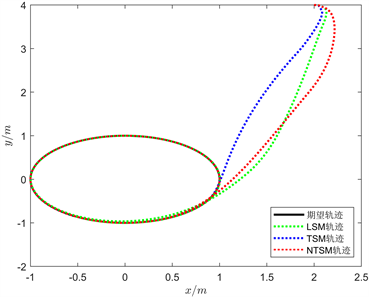Figure 1. The actual trajectory of vehicle system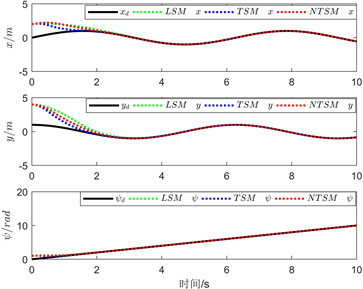Figure 2. Tracking trajectories for position and velocity comparison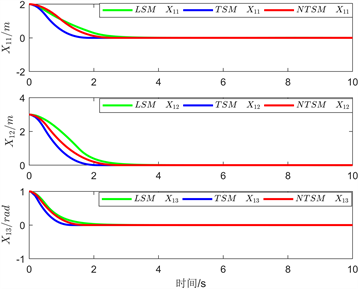Figure 3. Tracking trajectories for position and velocity comparison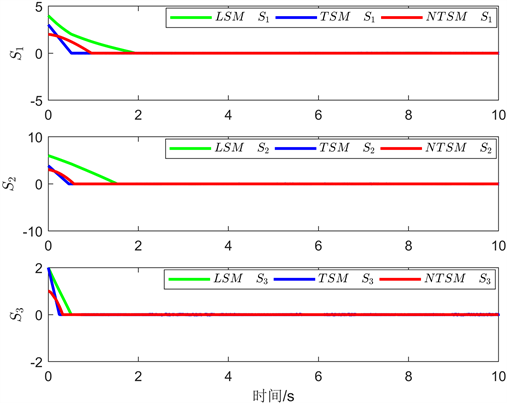Figure 4. Three types of sliding modes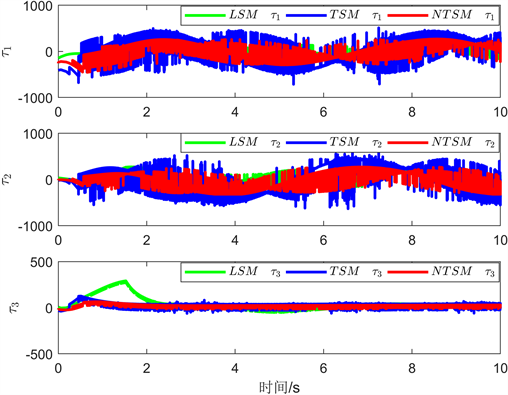Figure 5. Comparison diagram of control inputs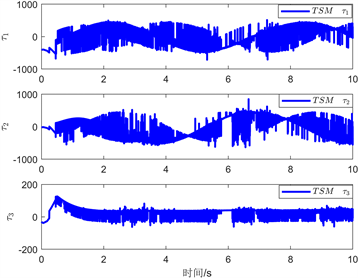Figure 6. Control inputs of TSM and NTSM

5. 结论